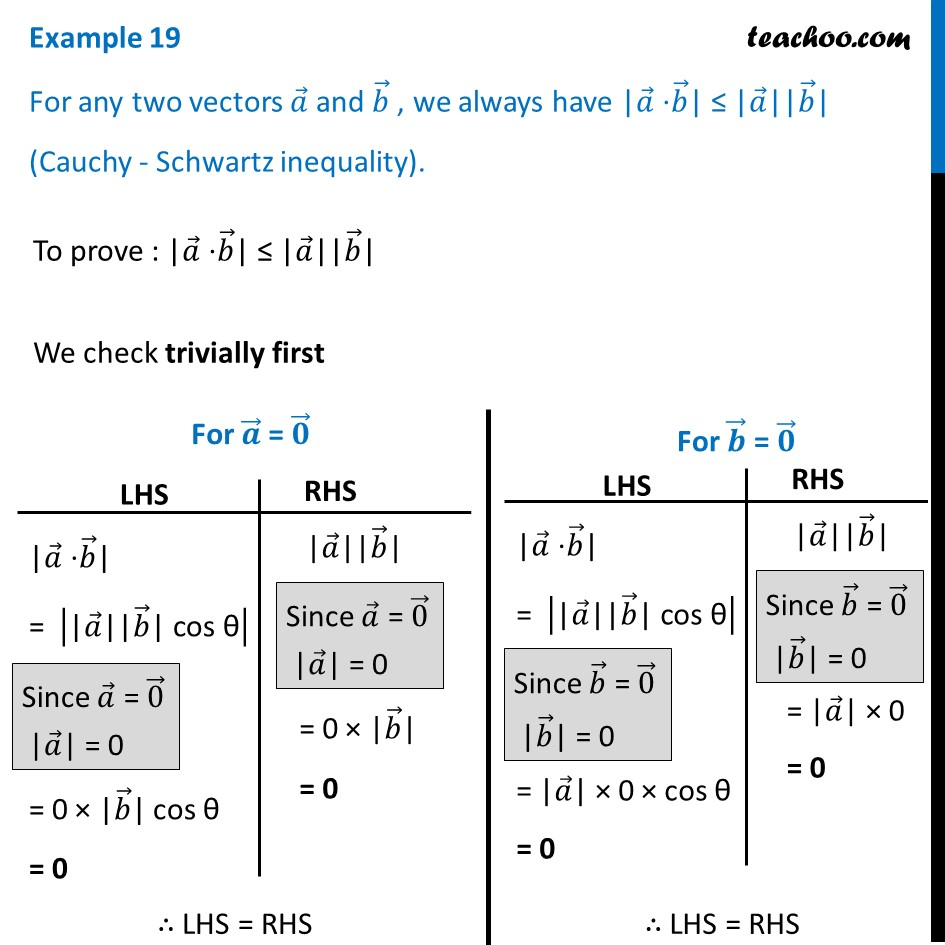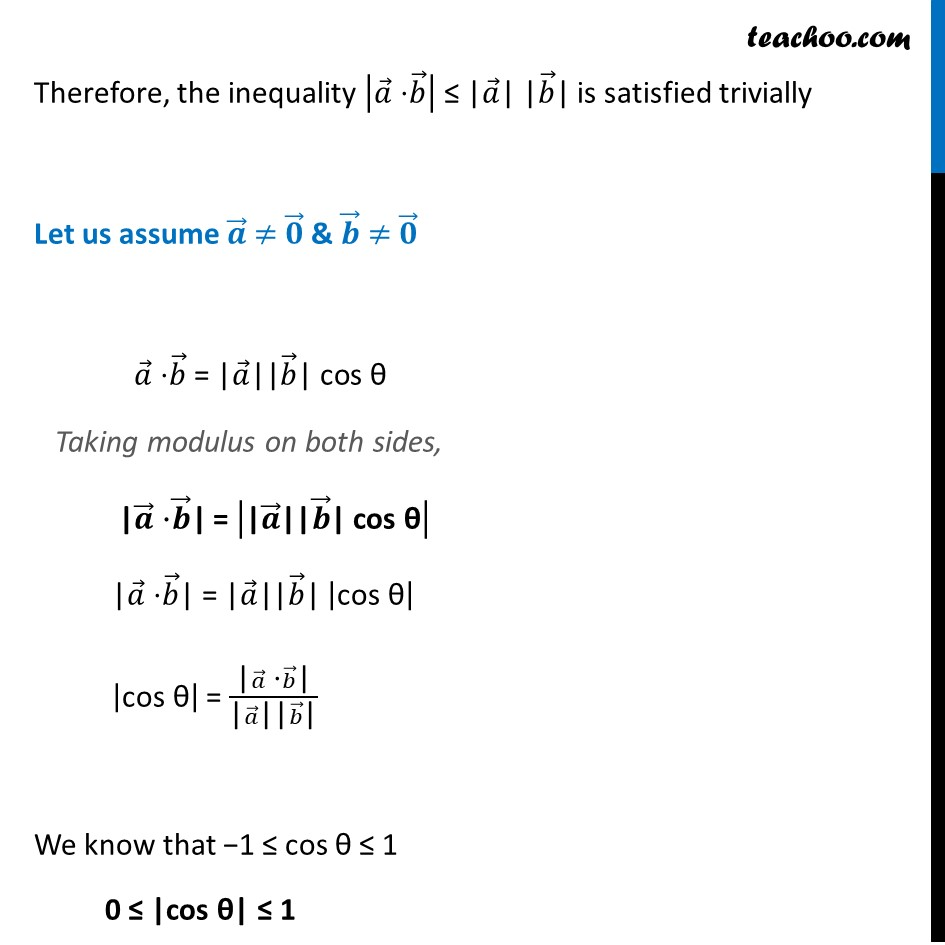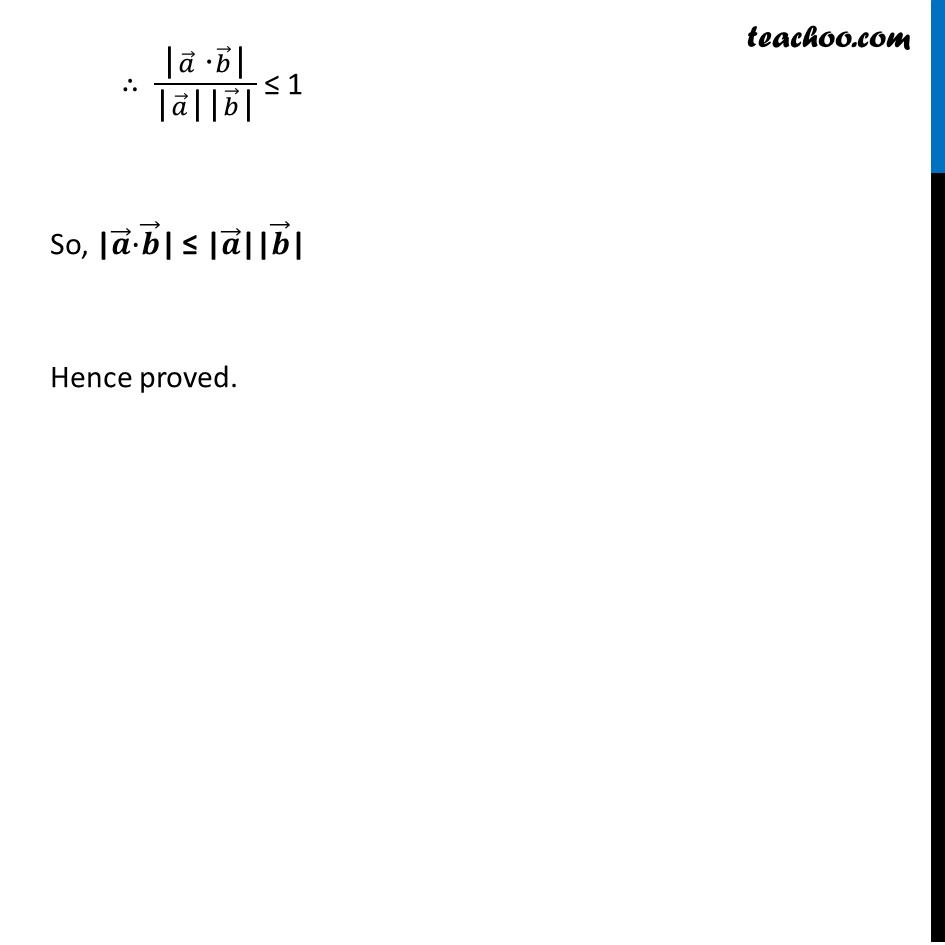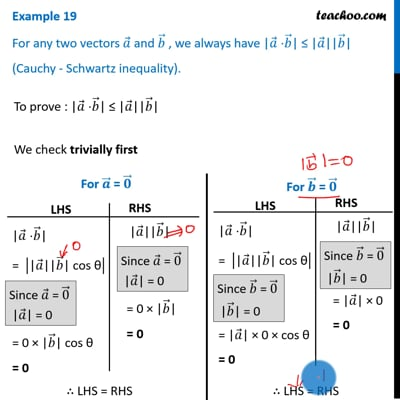Examples

Chapter 10 Class 12 Vector Algebra
Serial order wiseThis video is only available for Teachoo black users

Learn in your speed, with individual attention - Teachoo Maths 1-on-1 Class

### Transcript

Example 19 For any two vectors 𝑎 ⃗ and 𝑏 ⃗ , we always have |𝑎 ⃗ ⋅𝑏 ⃗| ≤ |𝑎 ⃗||𝑏 ⃗| (Cauchy - Schwartz inequality). To prove : |𝑎 ⃗ ⋅𝑏 ⃗| ≤ |𝑎 ⃗||𝑏 ⃗| We check trivially first |𝑎 ⃗ ⋅𝑏 ⃗| = |"|" 𝑎 ⃗"||" 𝑏 ⃗"| cos θ" | = 0 × |𝑏 ⃗| cos θ = 0 "|" 𝑎 ⃗"||" 𝑏 ⃗"|" = 0 × |𝑏 ⃗| = 0 |𝑎 ⃗ ⋅𝑏 ⃗| = |"|" 𝑎 ⃗"||" 𝑏 ⃗"| cos θ" | = |𝑎 ⃗| × 0 × cos θ = 0 |𝑎 ⃗ ⋅𝑏 ⃗| = |"|" 𝑎 ⃗"||" 𝑏 ⃗"| cos θ" | = |𝑎 ⃗| × 0 × cos θ = 0 "|" 𝑎 ⃗"||" 𝑏 ⃗"|" = |𝑎 ⃗| × 0 = 0 Therefore, the inequality |𝑎 ⃗" ⋅" 𝑏 ⃗ | ≤ |𝑎 ⃗| |𝑏 ⃗| is satisfied trivially Let us assume 𝒂 ⃗ ≠ 𝟎 ⃗ & 𝒃 ⃗ ≠ 𝟎 ⃗ 𝑎 ⃗ ⋅𝑏 ⃗ = "|" 𝑎 ⃗"||" 𝑏 ⃗"| cos θ" Taking modulus on both sides, |𝒂 ⃗ ⋅𝒃 ⃗| = |"|" 𝒂 ⃗"||" 𝒃 ⃗"| cos θ" | |𝑎 ⃗ ⋅𝑏 ⃗| = "|" 𝑎 ⃗"||" 𝑏 ⃗"|" |"cos θ" | |"cos θ" | = ("|" 𝑎 ⃗" ⋅" 𝑏 ⃗"|" )/("|" 𝑎 ⃗"||" 𝑏 ⃗"|" ) We know that −1 ≤ cos θ ≤ 1 0 ≤ "|cos θ|" ≤ 1 ∴ ("|" 𝑎 ⃗" ⋅" 𝑏 ⃗"|" )/("|" 𝑎 ⃗"||" 𝑏 ⃗"|" ) ≤ 1 So, |𝒂 ⃗⋅𝒃 ⃗| ≤ "|" 𝒂 ⃗"||" 𝒃 ⃗"|" Hence proved.